# Chemical Bonding I The Covalent Bond Copyright The

• Slides: 87
Download presentation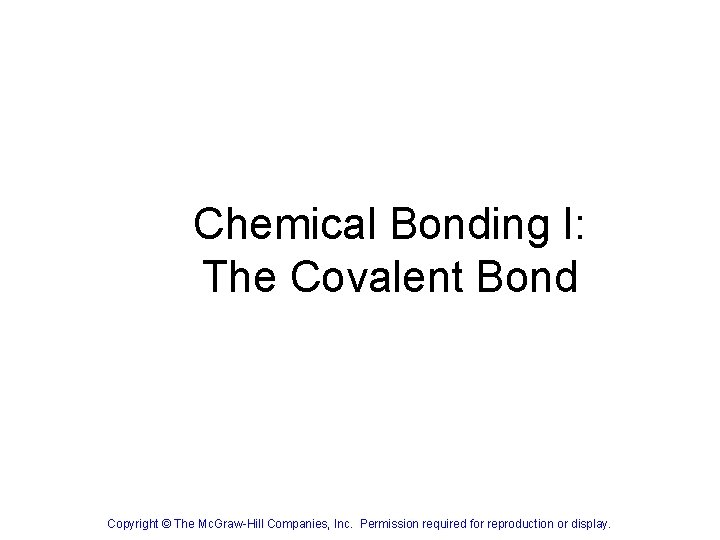Chemical Bonding I: The Covalent Bond Copyright © The Mc. Graw-Hill Companies, Inc. Permission required for reproduction or display.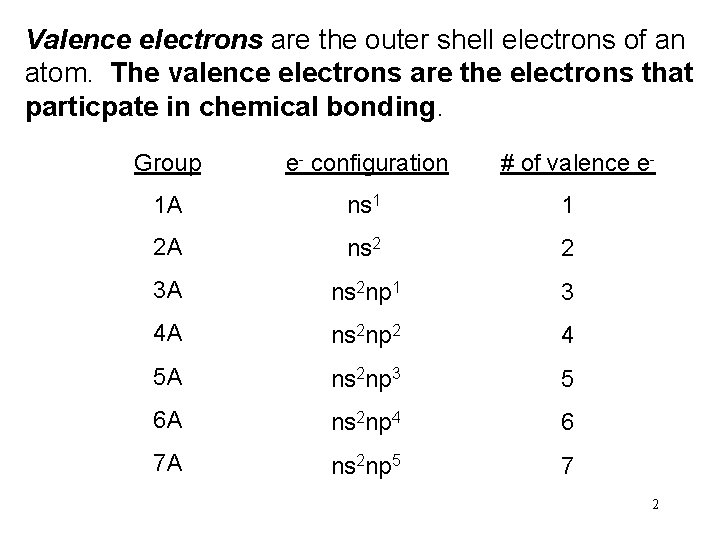Valence electrons are the outer shell electrons of an atom. The valence electrons are the electrons that particpate in chemical bonding. Group e- configuration # of valence e- 1 A ns 1 1 2 A ns 2 2 3 A ns 2 np 1 3 4 A ns 2 np 2 4 5 A ns 2 np 3 5 6 A ns 2 np 4 6 7 A ns 2 np 5 7 2Lewis Dot Symbols for the Representative Elements & Noble Gases 3The Ionic Bond Ionic bond: the electrostatic force that holds ions together in an ionic compound. Li + F 1 22 s 22 p 5 1 s 22 s 1 s Li Li. F e- + Li+ F 1 s 22 s 22 p 6 [He] [Ne] Li+ + e- F F - Li+ F - 4Electrostatic (Lattice) Energy Lattice energy (U) (LE) of an ionic compound is the energy required to break apart the ions in their lattice arrangement into the ions in the gas phase. For example the energy to take Na. Cl from its lattice arrangement into the gas phase ions would be represented by Na. Cl(s) ---> Na+(g) + Cl-(g) 5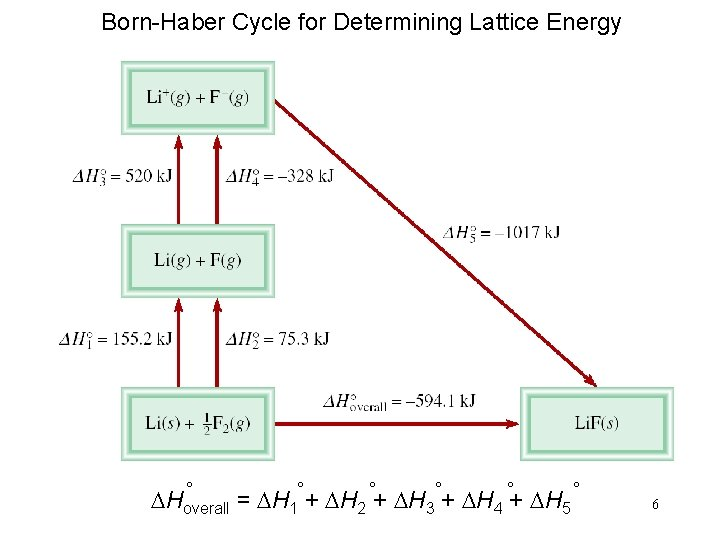Born-Haber Cycle for Determining Lattice Energy ° ° ° DHoverall = DH 1° + DH 2 + DH 3 4 5 67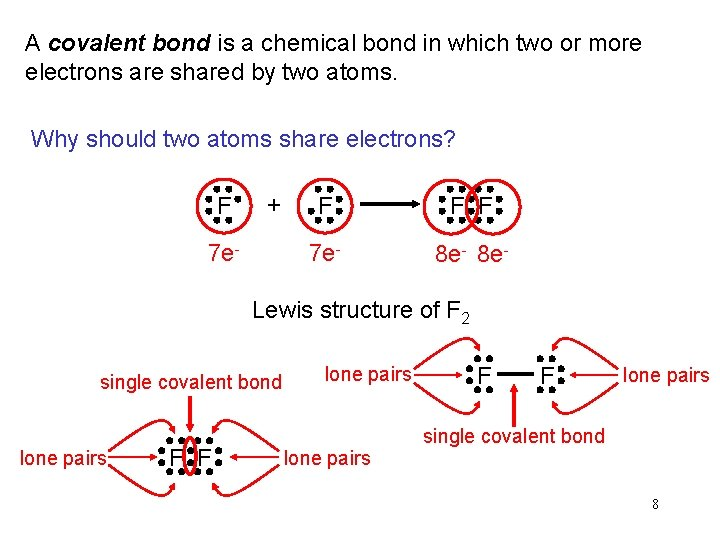A covalent bond is a chemical bond in which two or more electrons are shared by two atoms. Why should two atoms share electrons? F + 7 e- F F F 7 e- 8 e- Lewis structure of F 2 single covalent bond lone pairs F F lone pairs single covalent bond 8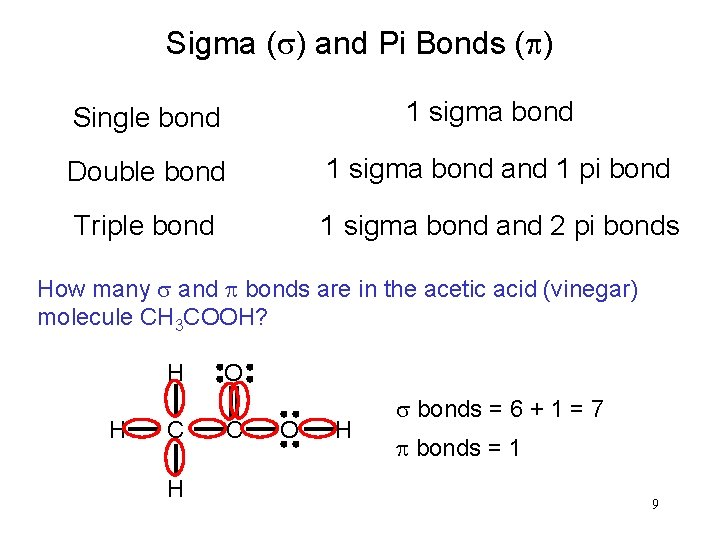Sigma (s) and Pi Bonds (p) 1 sigma bond Single bond Double bond 1 sigma bond and 1 pi bond Triple bond 1 sigma bond and 2 pi bonds How many s and p bonds are in the acetic acid (vinegar) molecule CH 3 COOH? H C H O H C O H s bonds = 6 + 1 = 7 p bonds = 1 9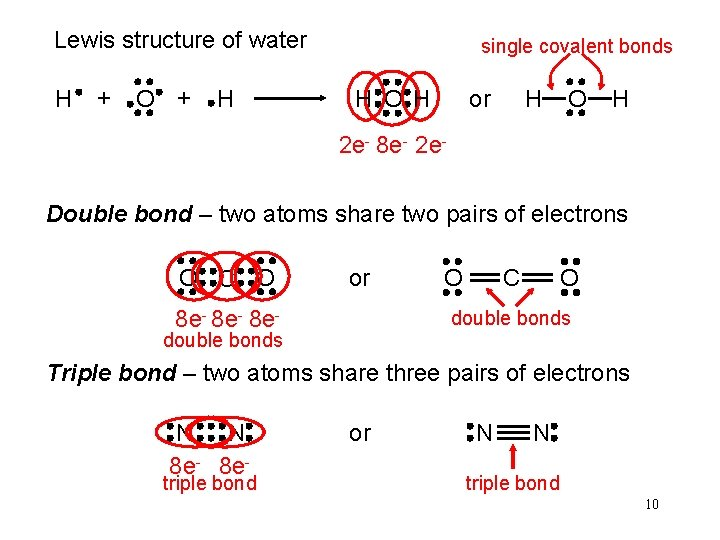Lewis structure of water H + O + H single covalent bonds H O H or H O H 2 e- 8 e- 2 e. Double bond – two atoms share two pairs of electrons O C O or 8 e- 8 e- O O C double bonds Triple bond – two atoms share three pairs of electrons N N 8 e- triple bond or N N triple bond 10Lengths of Covalent Bonds Bond Lengths Triple bond < Double Bond < Single Bond 11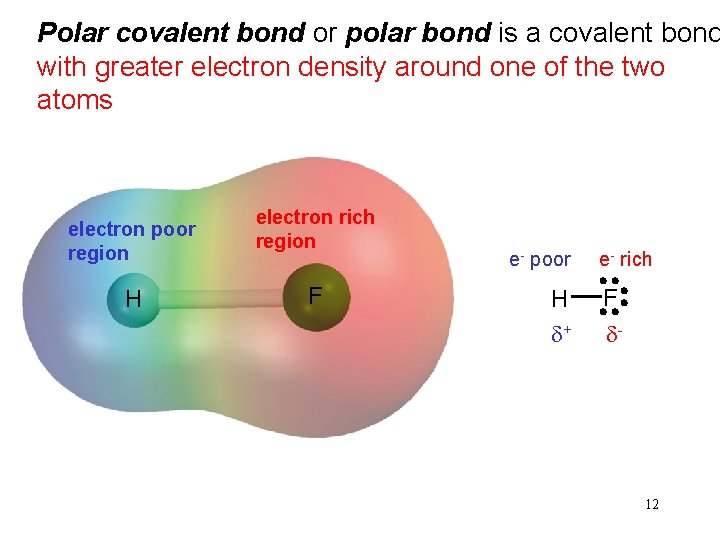Polar covalent bond or polar bond is a covalent bond with greater electron density around one of the two atoms electron poor region H electron rich region F e- poor H d+ e- rich F d- 12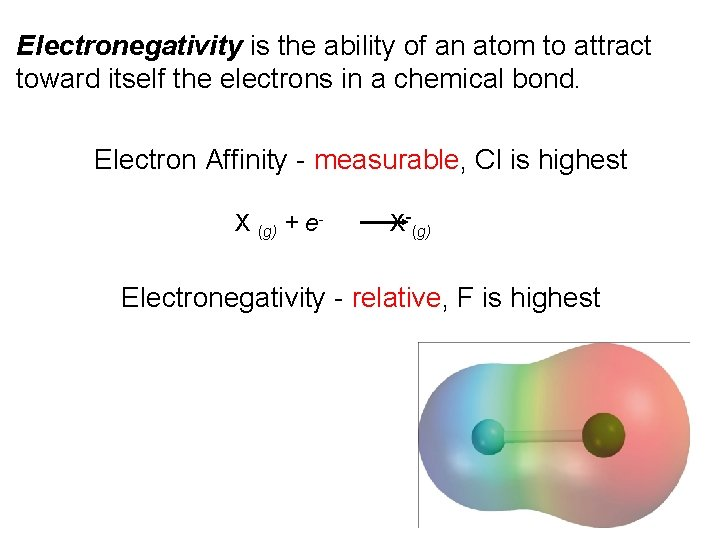Electronegativity is the ability of an atom to attract toward itself the electrons in a chemical bond. Electron Affinity - measurable, Cl is highest X (g) + e- X-(g) Electronegativity - relative, F is highest 13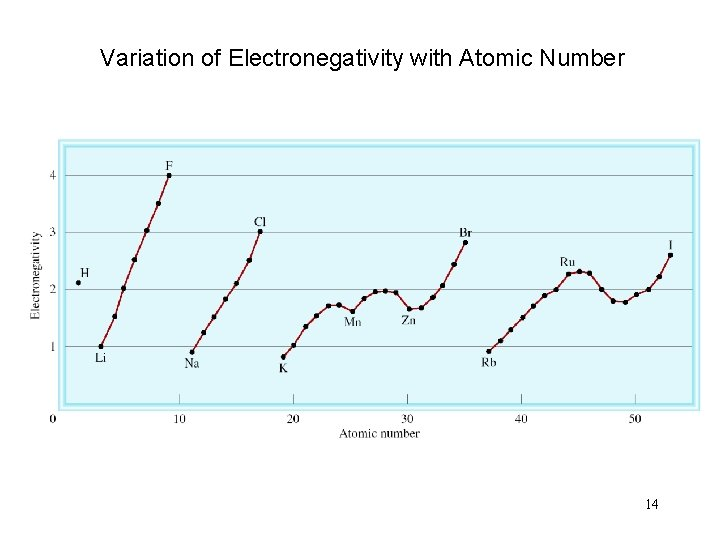Variation of Electronegativity with Atomic Number 14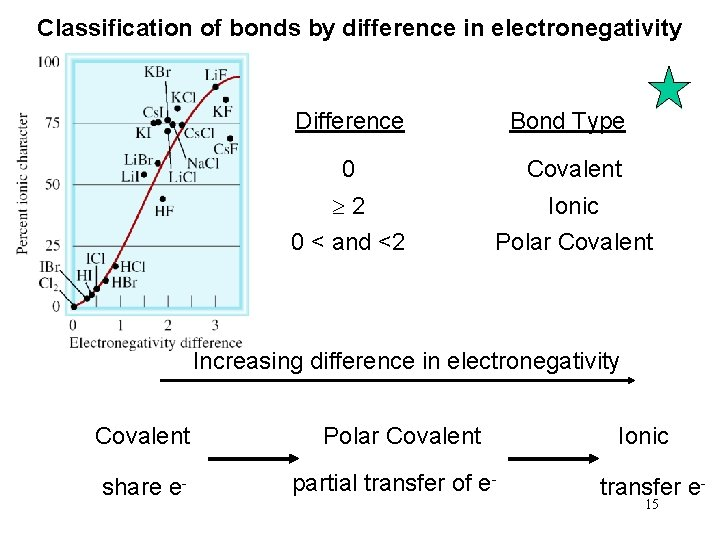Classification of bonds by difference in electronegativity Difference Bond Type 0 Covalent 2 0 < and <2 Ionic Polar Covalent Increasing difference in electronegativity Covalent share e- Polar Covalent partial transfer of e- Ionic transfer e 15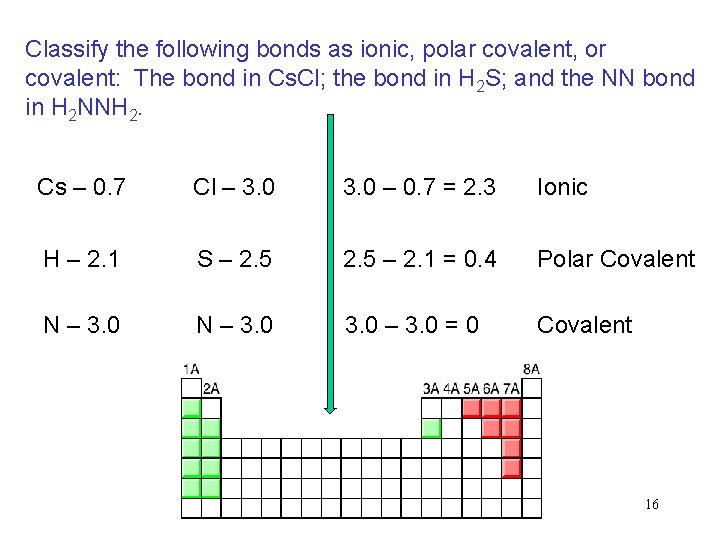Classify the following bonds as ionic, polar covalent, or covalent: The bond in Cs. Cl; the bond in H 2 S; and the NN bond in H 2 NNH 2. Cs – 0. 7 Cl – 3. 0 – 0. 7 = 2. 3 Ionic H – 2. 1 S – 2. 5 – 2. 1 = 0. 4 Polar Covalent N – 3. 0 – 3. 0 = 0 Covalent 16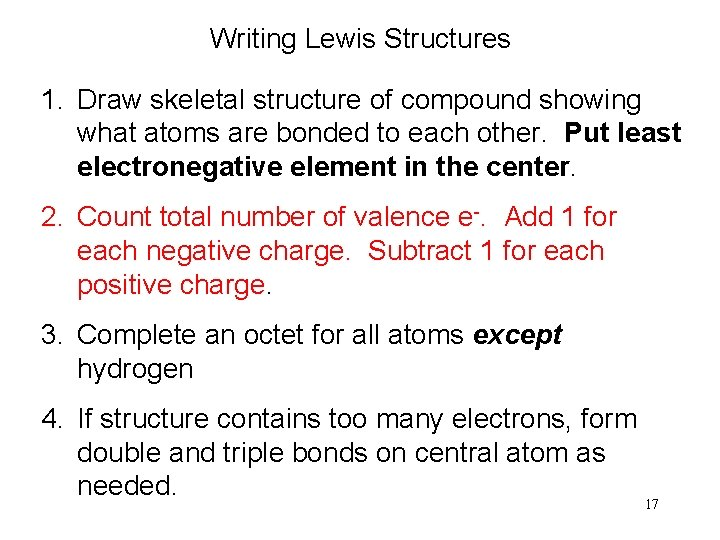Writing Lewis Structures 1. Draw skeletal structure of compound showing what atoms are bonded to each other. Put least electronegative element in the center. 2. Count total number of valence e-. Add 1 for each negative charge. Subtract 1 for each positive charge. 3. Complete an octet for all atoms except hydrogen 4. If structure contains too many electrons, form double and triple bonds on central atom as needed. 17Write the Lewis structure of nitrogen trifluoride (NF 3). Step 1 – N is less electronegative than F, put N in center Step 2 – Count valence electrons N - 5 (2 s 22 p 3) and F - 7 (2 s 22 p 5) 5 + (3 x 7) = 26 valence electrons Step 3 – Draw single bonds between N and F atoms and complete octets on N and F atoms. Step 4 - Check, are # of e- in structure equal to number of valence e- ? 3 single bonds (3 x 2) + 10 lone pairs (10 x 2) = 26 valence electrons F N F F 18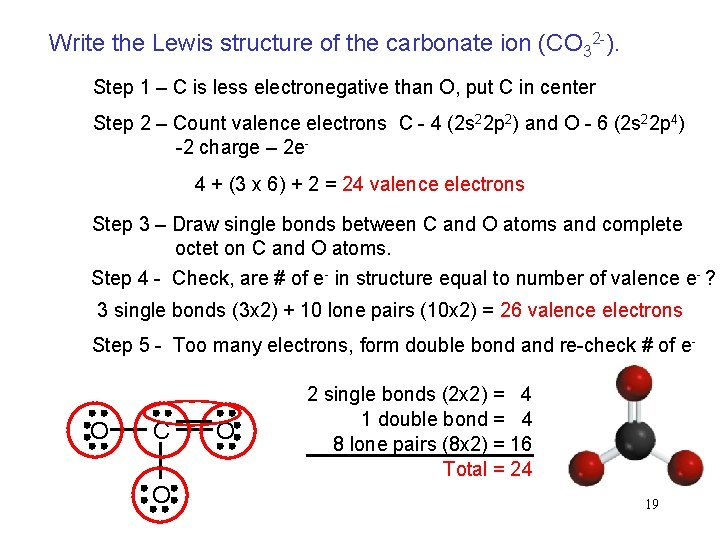Write the Lewis structure of the carbonate ion (CO 32 -). Step 1 – C is less electronegative than O, put C in center Step 2 – Count valence electrons C - 4 (2 s 22 p 2) and O - 6 (2 s 22 p 4) -2 charge – 2 e- 4 + (3 x 6) + 2 = 24 valence electrons Step 3 – Draw single bonds between C and O atoms and complete octet on C and O atoms. Step 4 - Check, are # of e- in structure equal to number of valence e- ? 3 single bonds (3 x 2) + 10 lone pairs (10 x 2) = 26 valence electrons Step 5 - Too many electrons, form double bond and re-check # of e- O C O O 2 single bonds (2 x 2) = 4 1 double bond = 4 8 lone pairs (8 x 2) = 16 Total = 24 19A resonance structure is one of two or more Lewis structures for a single molecule that cannot be represented accurately by only one Lewis structure. O O + O - - O + O O What are the resonance structures of the carbonate (CO 32 -) ion? - O C O O - - - O C O O - 20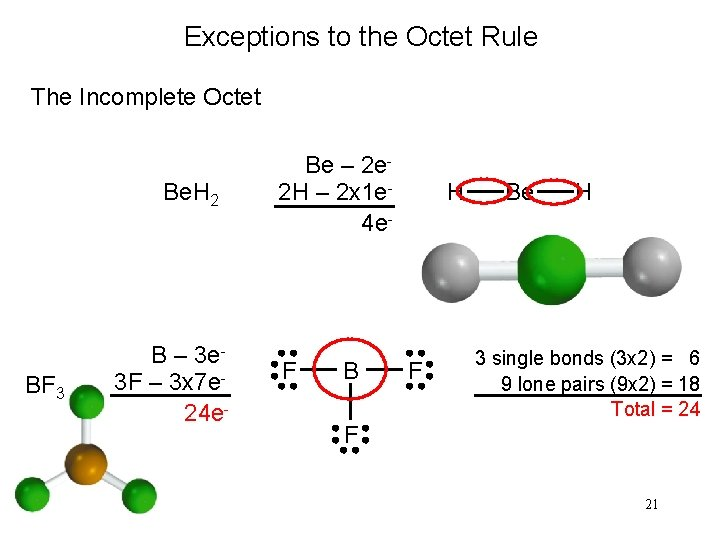Exceptions to the Octet Rule The Incomplete Octet Be. H 2 BF 3 B – 3 e 3 F – 3 x 7 e 24 e- Be – 2 e 2 H – 2 x 1 e 4 e- F B H F Be H 3 single bonds (3 x 2) = 6 9 lone pairs (9 x 2) = 18 Total = 24 F 21Exceptions to the Octet Rule Odd-Electron Molecules NO N – 5 e. O – 6 e 11 e- N O The Expanded Octet (central atom with principal quantum number n > 2) SF 6 S – 6 e 6 F – 42 e 48 e- F F F S F F F 6 single bonds (6 x 2) = 12 18 lone pairs (18 x 2) = 36 Total = 48 22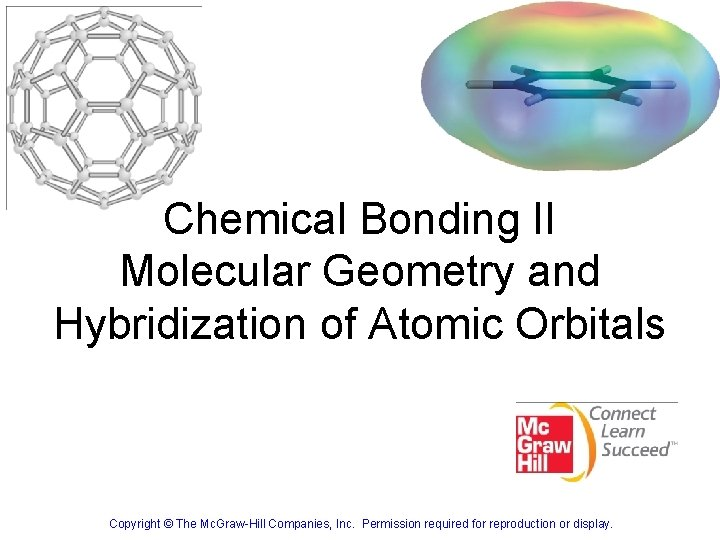Chemical Bonding II Molecular Geometry and Hybridization of Atomic Orbitals 23 Copyright © The Mc. Graw-Hill Companies, Inc. Permission required for reproduction or display.Valence shell electron pair repulsion (VSEPR) model: Predict the geometry of the molecule from the electrostatic repulsions between the electron (bonding and nonbonding) pairs. Class # of atoms bonded to central atom # lone pairs on central atom Arrangement of electron pairs Molecular Geometry AB 2 2 0 linear B B 240 lone pairs on central atom Cl Be Cl 2 atoms bonded to central atom 25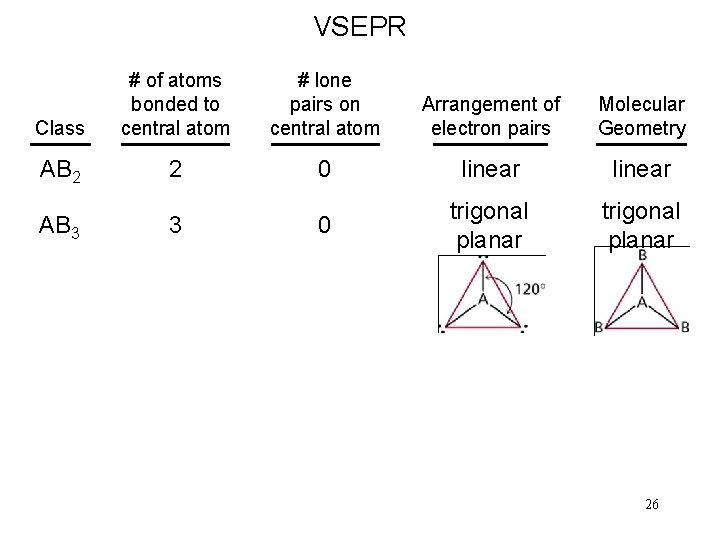VSEPR Class # of atoms bonded to central atom # lone pairs on central atom Arrangement of electron pairs Molecular Geometry AB 2 2 0 linear 0 trigonal planar AB 3 3 2627VSEPR Class # of atoms bonded to central atom # lone pairs on central atom Arrangement of electron pairs Molecular Geometry AB 2 2 0 linear trigonal planar AB 3 3 0 trigonal planar AB 4 4 0 tetrahedral 28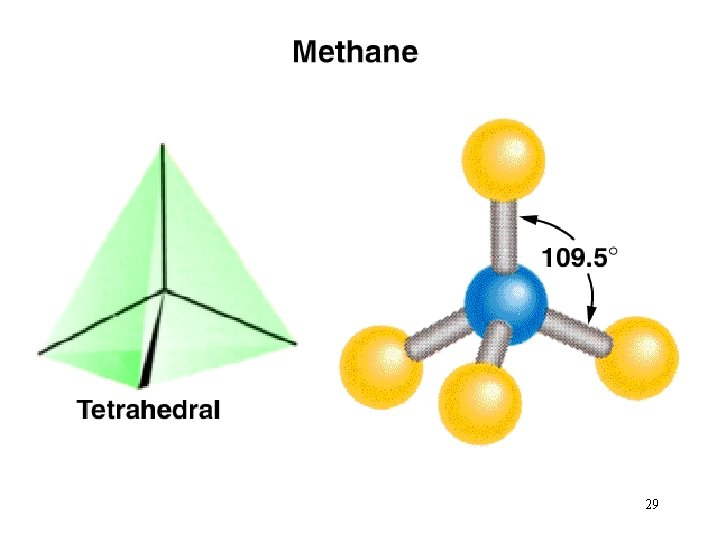29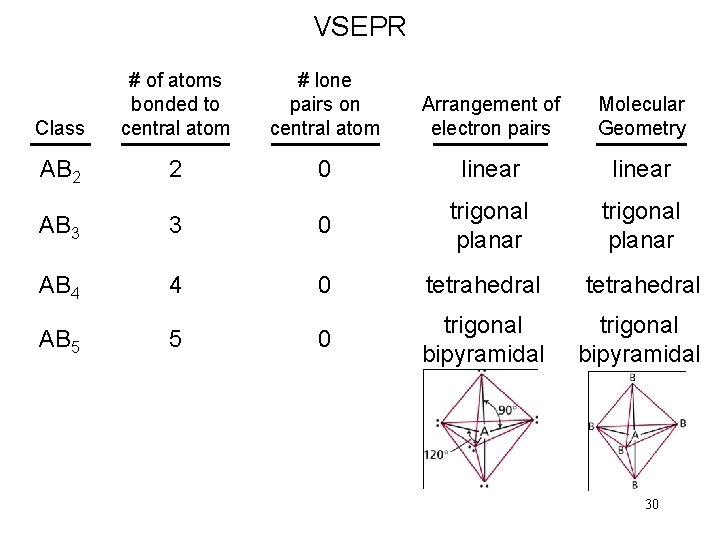VSEPR Class # of atoms bonded to central atom # lone pairs on central atom Arrangement of electron pairs Molecular Geometry AB 2 2 0 linear trigonal planar AB 3 3 0 trigonal planar AB 4 4 0 tetrahedral AB 5 5 0 trigonal bipyramidal 30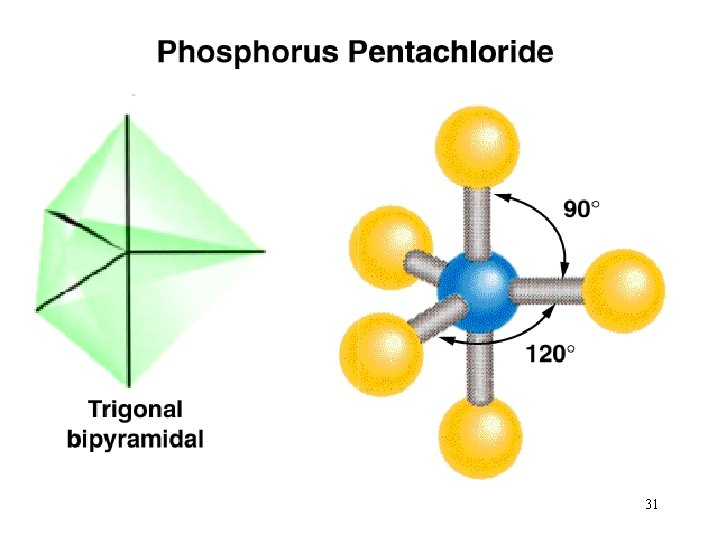31VSEPR Class # of atoms bonded to central atom # lone pairs on central atom Arrangement of electron pairs Molecular Geometry AB 2 2 0 linear trigonal planar AB 3 3 0 trigonal planar AB 4 4 0 tetrahedral AB 5 5 0 trigonal bipyramidal AB 6 6 0 octahedral 32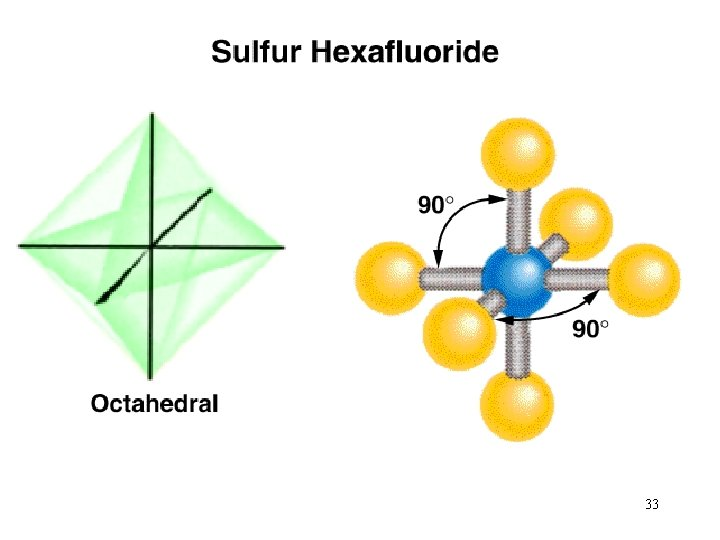33Predicting Molecular Geometry 1. Draw Lewis structure for molecule. 2. Count number of lone pairs on the central atom and number of atoms bonded to the central atom. 3. Use VSEPR to predict the geometry of the molecule. What are the molecular geometries of SO 2 and SF 4? O S AB 2 E bent F O F S F AB 4 E F distorted tetrahedron 34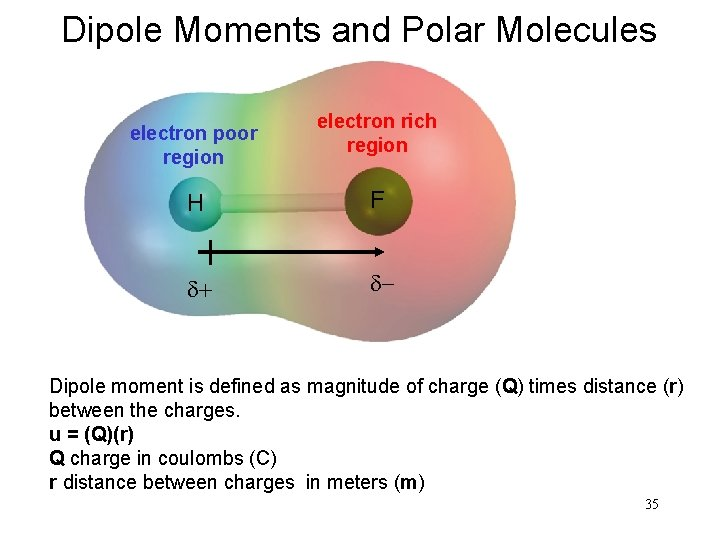Dipole Moments and Polar Molecules electron poor region electron rich region H F d+ d- Dipole moment is defined as magnitude of charge (Q) times distance (r) between the charges. u = (Q)(r) Q charge in coulombs (C) r distance between charges in meters (m) 35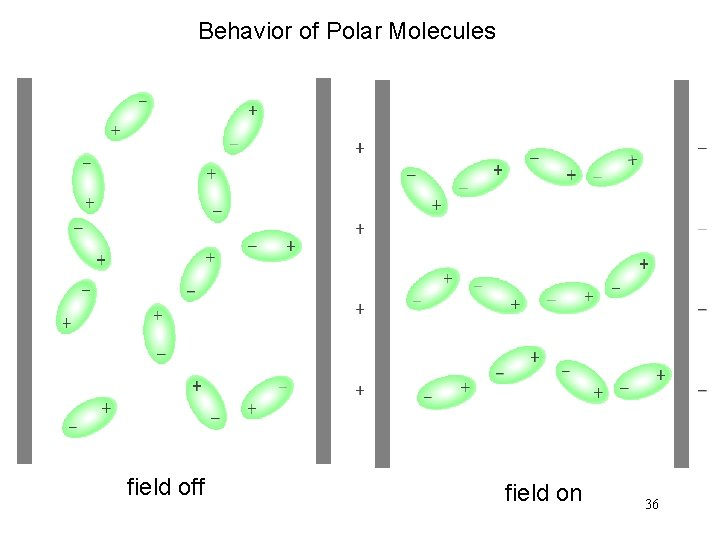Behavior of Polar Molecules field off field on 36Which of the following molecules have a dipole moment? H 2 O, CO 2, SO 2, and CF 4 O H H dipole moment polar molecule S O O dipole moment polar molecule F O C O no dipole moment nonpolar molecule C F F F no dipole moment nonpolar molecule 37Does CH 2 Cl 2 have a dipole moment? 3839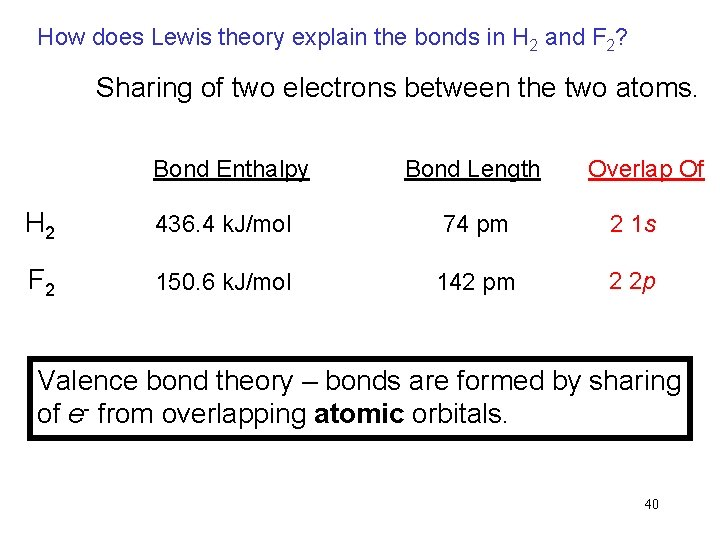How does Lewis theory explain the bonds in H 2 and F 2? Sharing of two electrons between the two atoms. Bond Enthalpy Bond Length Overlap Of H 2 436. 4 k. J/mol 74 pm 2 1 s F 2 150. 6 k. J/mol 142 pm 2 2 p Valence bond theory – bonds are formed by sharing of e- from overlapping atomic orbitals. 40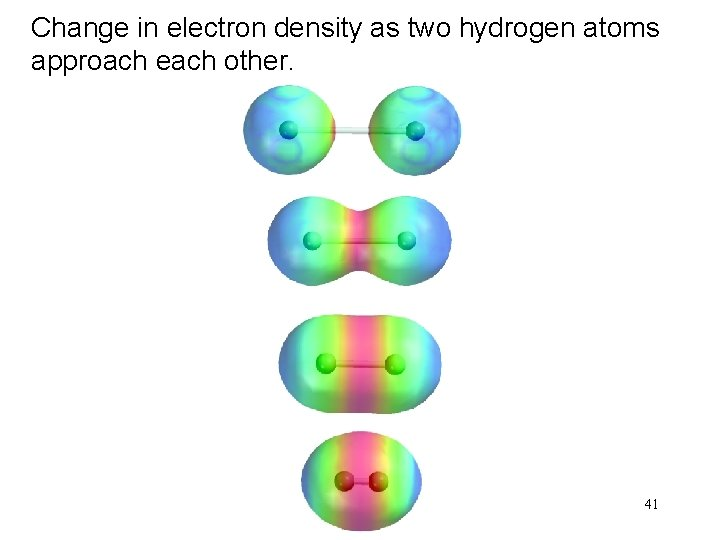Change in electron density as two hydrogen atoms approach each other. 41Valence Bond Theory and NH 3 N – 1 s 22 p 3 3 H – 1 s 1 If the bonds form from overlap of 3 2 p orbitals on nitrogen with the 1 s orbital on each hydrogen atom, what would the molecular geometry of NH 3 be? If use the 3 2 p orbitals predict 90 o Actual H-N-H bond angle is 107. 3 o 42Hybridization – mixing of two or more atomic orbitals to form a new set of hybrid orbitals. 1. Mix at least 2 nonequivalent atomic orbitals (e. g. s and p). Hybrid orbitals have very different shape from original atomic orbitals. 2. Number of hybrid orbitals is equal to number of pure atomic orbitals used in the hybridization process. 3. Covalent bonds are formed by: a. Overlap of hybrid orbitals with atomic orbitals b. Overlap of hybrid orbitals with other hybrid orbitals 43Formation of sp 3 Hybrid Orbitals 44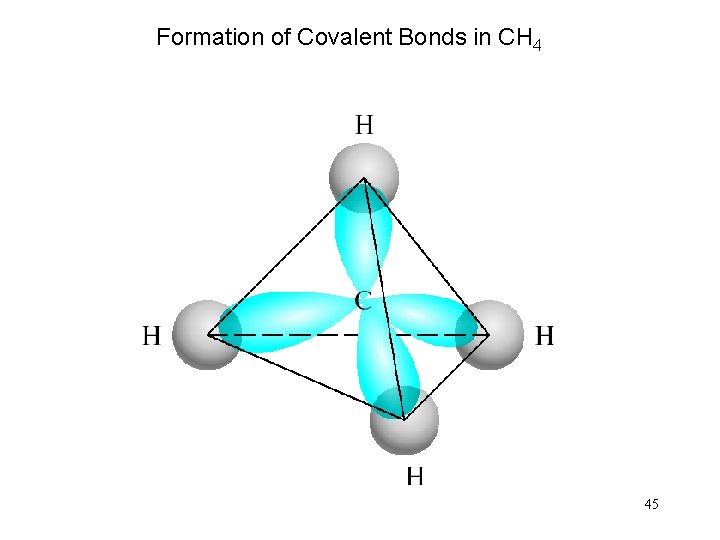Formation of Covalent Bonds in CH 4 45Formation of sp Hybrid Orbitals 46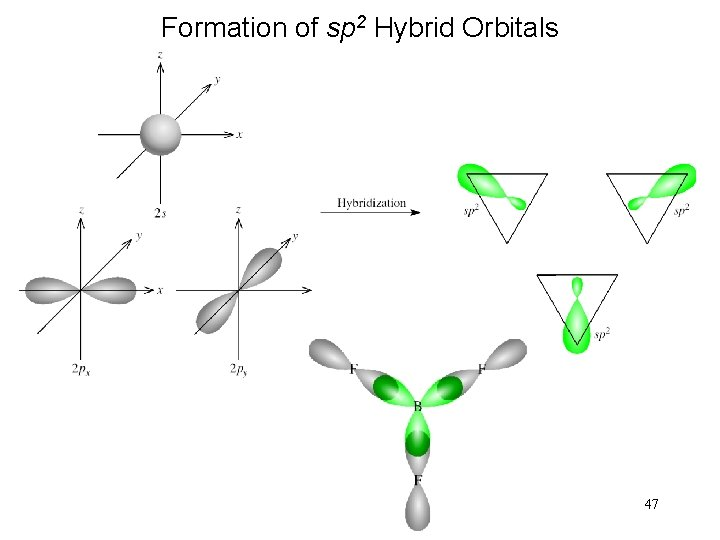Formation of sp 2 Hybrid Orbitals 47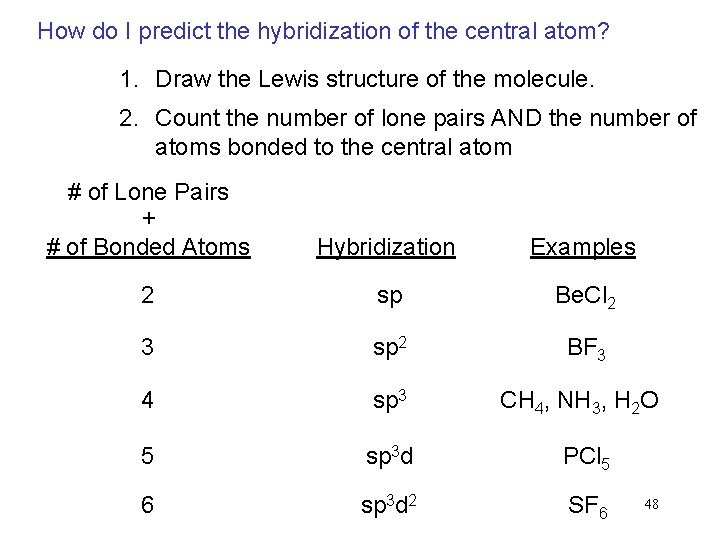How do I predict the hybridization of the central atom? 1. Draw the Lewis structure of the molecule. 2. Count the number of lone pairs AND the number of atoms bonded to the central atom # of Lone Pairs + # of Bonded Atoms Hybridization Examples 2 sp Be. Cl 2 3 sp 2 BF 3 4 sp 3 CH 4, NH 3, H 2 O 5 sp 3 d PCl 5 6 sp 3 d 2 SF 6 48Stoichiometry Copyright © The Mc. Graw-Hill Companies, Inc. Permission required for reproduction or display.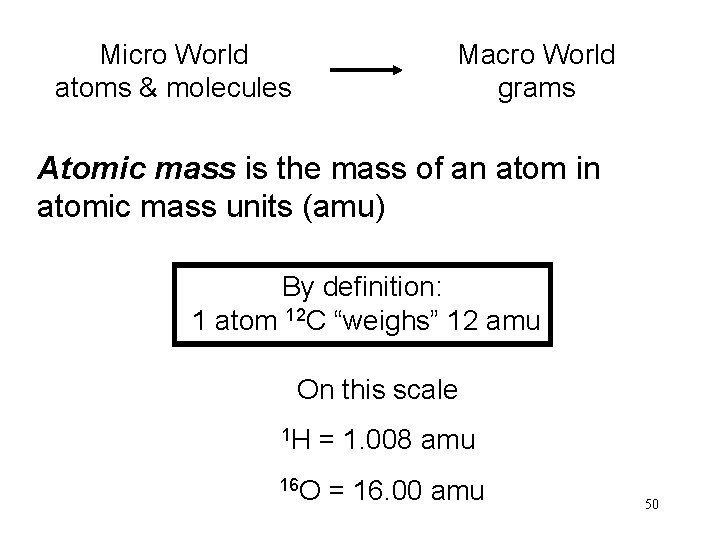Micro World atoms & molecules Macro World grams Atomic mass is the mass of an atom in atomic mass units (amu) By definition: 1 atom 12 C “weighs” 12 amu On this scale 1 H = 1. 008 amu 16 O = 16. 00 amu 50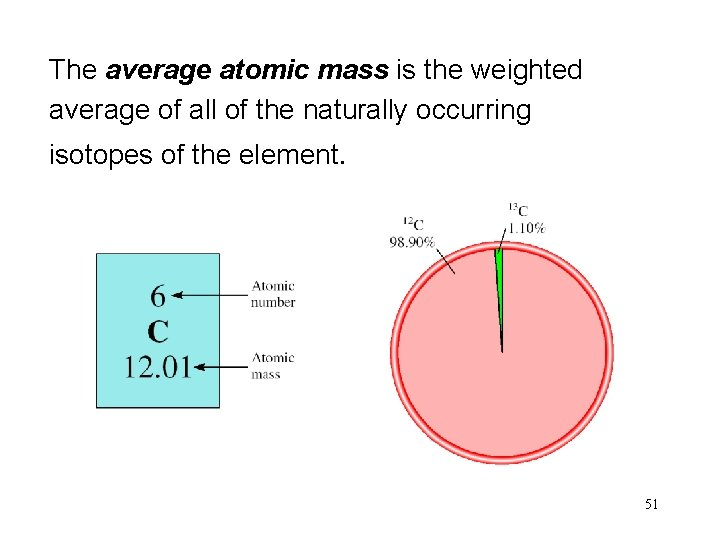The average atomic mass is the weighted average of all of the naturally occurring isotopes of the element. 51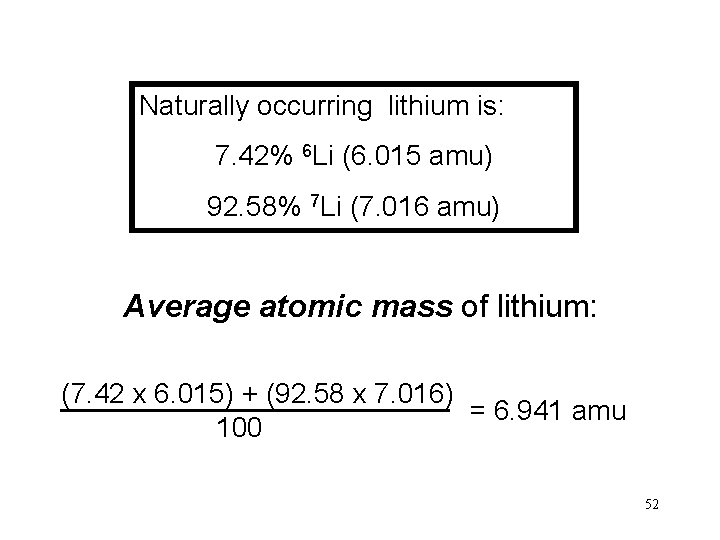Naturally occurring lithium is: 7. 42% 6 Li (6. 015 amu) 92. 58% 7 Li (7. 016 amu) Average atomic mass of lithium: (7. 42 x 6. 015) + (92. 58 x 7. 016) = 6. 941 amu 100 52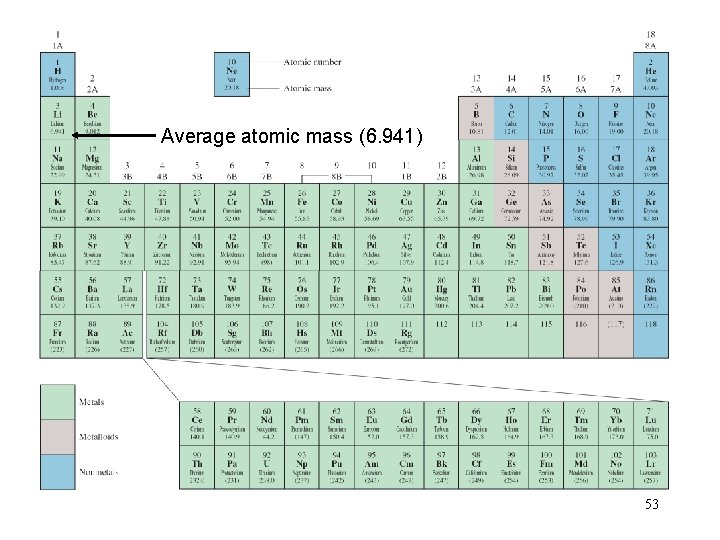Average atomic mass (6. 941) 53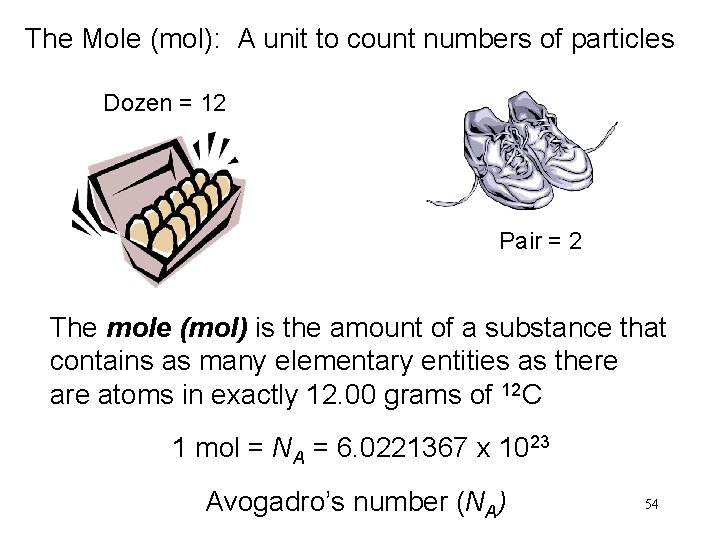The Mole (mol): A unit to count numbers of particles Dozen = 12 Pair = 2 The mole (mol) is the amount of a substance that contains as many elementary entities as there atoms in exactly 12. 00 grams of 12 C 1 mol = NA = 6. 0221367 x 1023 Avogadro’s number (NA) 54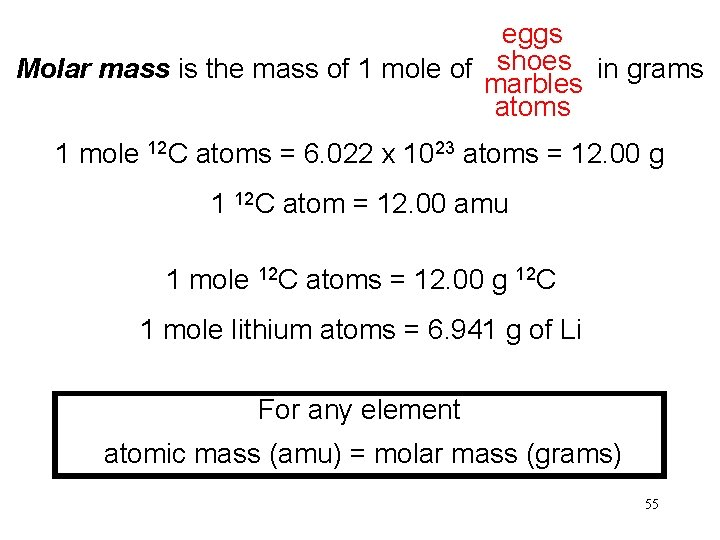eggs shoes Molar mass is the mass of 1 mole of in grams marbles atoms 1 mole 12 C atoms = 6. 022 x 1023 atoms = 12. 00 g 1 12 C atom = 12. 00 amu 1 mole 12 C atoms = 12. 00 g 12 C 1 mole lithium atoms = 6. 941 g of Li For any element atomic mass (amu) = molar mass (grams) 55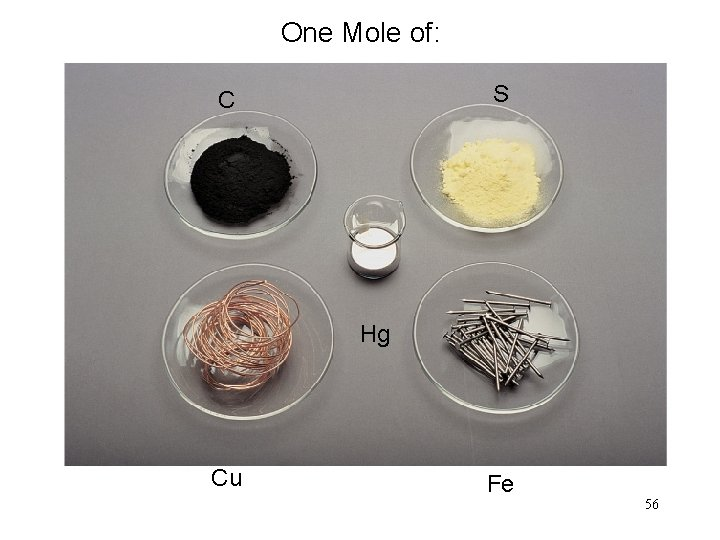One Mole of: S C Hg Cu Fe 56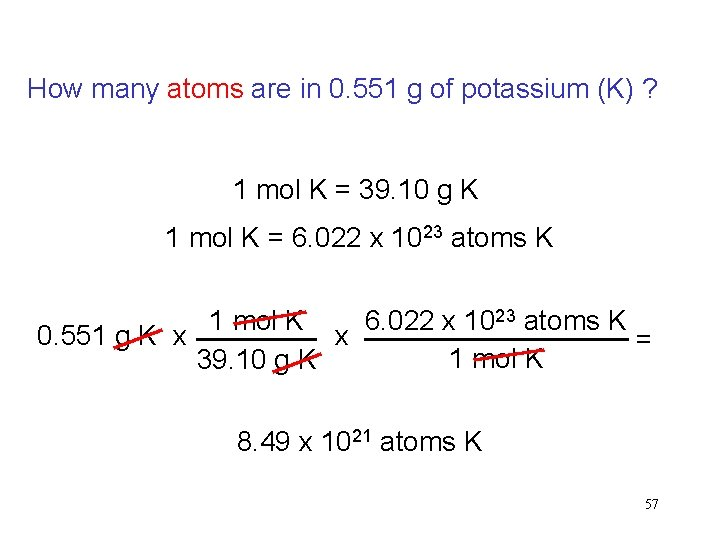How many atoms are in 0. 551 g of potassium (K) ? 1 mol K = 39. 10 g K 1 mol K = 6. 022 x 1023 atoms K 1 mol K 6. 022 x 1023 atoms K 0. 551 g K x x = 1 mol K 39. 10 g K 8. 49 x 1021 atoms K 57Molecular mass (or molecular weight) is the sum of the atomic masses (in amu) in a molecule. 1 S SO 2 2 O SO 2 32. 07 amu + 2 x 16. 00 amu 64. 07 amu For any molecule molecular mass (amu) = molar mass (grams) 1 molecule SO 2 = 64. 07 amu 1 mole SO 2 = 64. 07 g SO 2 58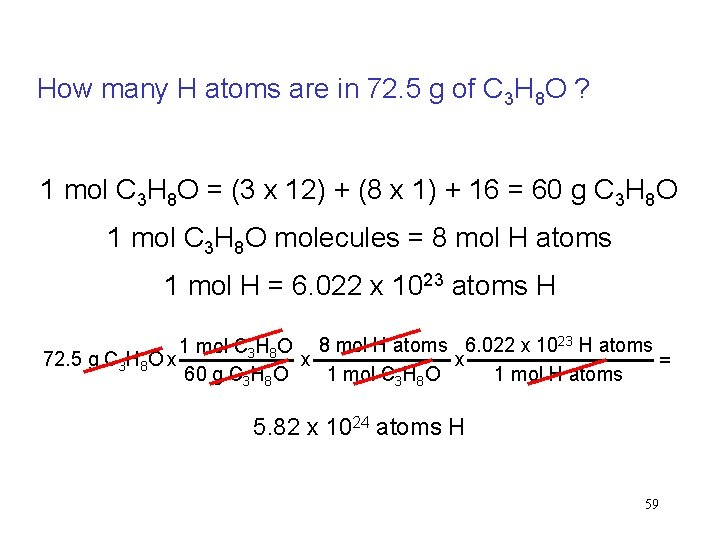How many H atoms are in 72. 5 g of C 3 H 8 O ? 1 mol C 3 H 8 O = (3 x 12) + (8 x 1) + 16 = 60 g C 3 H 8 O 1 mol C 3 H 8 O molecules = 8 mol H atoms 1 mol H = 6. 022 x 1023 atoms H 1 mol C 3 H 8 O 8 mol H atoms 6. 022 x 1023 H atoms 72. 5 g C 3 H 8 O x x x = 1 mol C 3 H 8 O 1 mol H atoms 60 g C 3 H 8 O 5. 82 x 1024 atoms H 59Formula mass is the sum of the atomic masses (in amu) in a formula unit of an ionic compound. 1 Na Na. Cl 22. 99 amu 1 Cl + 35. 45 amu Na. Cl 58. 44 amu For any ionic compound formula mass (amu) = molar mass (grams) 1 formula unit Na. Cl = 58. 44 amu 1 mole Na. Cl = 58. 44 g Na. Cl 60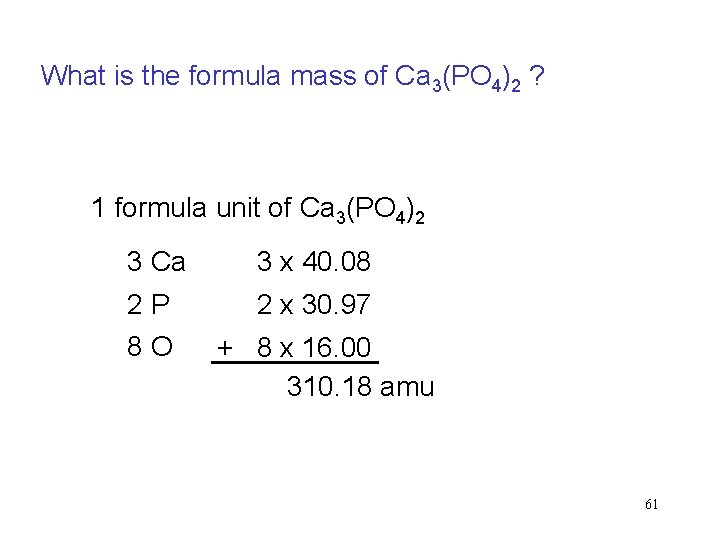What is the formula mass of Ca 3(PO 4)2 ? 1 formula unit of Ca 3(PO 4)2 3 Ca 3 x 40. 08 2 P 8 O 2 x 30. 97 + 8 x 16. 00 310. 18 amu 61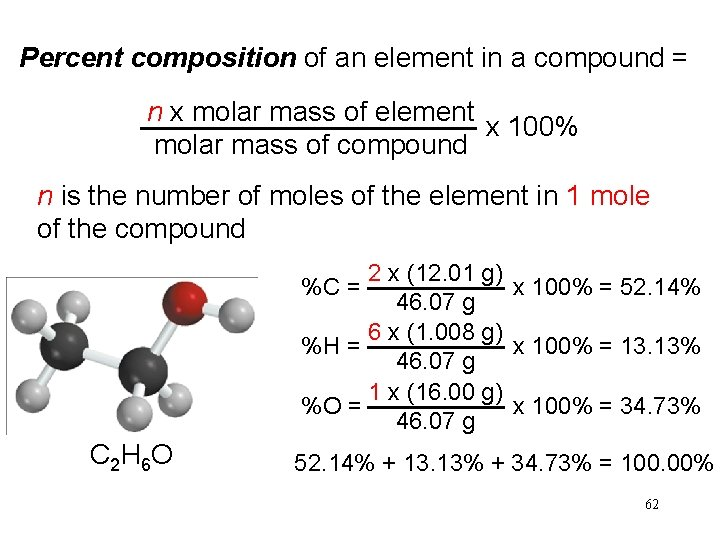Percent composition of an element in a compound = n x molar mass of element x 100% molar mass of compound n is the number of moles of the element in 1 mole of the compound 2 x (12. 01 g) x 100% = 52. 14% 46. 07 g 6 x (1. 008 g) %H = x 100% = 13. 13% 46. 07 g 1 x (16. 00 g) %O = x 100% = 34. 73% 46. 07 g %C = C 2 H 6 O 52. 14% + 13. 13% + 34. 73% = 100. 00% 62Percent Composition and Empirical Formulas Determine the empirical formula of a compound that has the following percent composition by mass: K 24. 75, Mn 34. 77, O 40. 51 percent. 1 mol K n. K = 24. 75 g K x = 0. 6330 mol K 39. 10 g K 1 mol Mn n. Mn = 34. 77 g Mn x = 0. 6329 mol Mn 54. 94 g Mn n. O = 40. 51 g O x 1 mol O = 2. 532 mol O 16. 00 g O 63Percent Composition and Empirical Formulas n. K = 0. 6330, n. Mn = 0. 6329, n. O = 2. 532 0. 6330 ~ K : ~ 1. 0 0. 6329 Mn : 0. 6329 = 1. 0 0. 6329 2. 532 ~ O : ~ 4. 0 0. 6329 KMn. O 4 64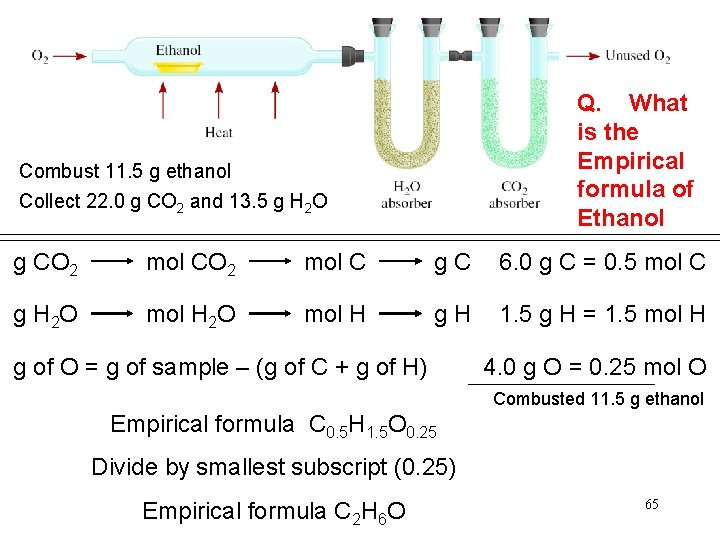Q. What is the Empirical formula of Ethanol Combust 11. 5 g ethanol Collect 22. 0 g CO 2 and 13. 5 g H 2 O g CO 2 mol C g C 6. 0 g C = 0. 5 mol C g H 2 O mol H g H 1. 5 g H = 1. 5 mol H g of O = g of sample – (g of C + g of H) 4. 0 g O = 0. 25 mol O Combusted 11. 5 g ethanol Empirical formula C 0. 5 H 1. 5 O 0. 25 Divide by smallest subscript (0. 25) Empirical formula C 2 H 6 O 65A process in which one or more substances is changed into one or more new substances is a chemical reaction A chemical equation uses chemical symbols to show what happens during a chemical reaction reactants products 3 ways of representing the reaction of H 2 with O 2 to form H 2 O 66How to “Read” Chemical Equations 2 Mg + O 2 2 Mg. O 2 atoms Mg + 1 molecule O 2 makes 2 formula units Mg. O 2 moles Mg + 1 mole O 2 makes 2 moles Mg. O 48. 6 grams Mg + 32. 0 grams O 2 makes 80. 6 g Mg. O NOT 2 grams Mg + 1 gram O 2 makes 2 g Mg. O 67Balancing Chemical Equations 1. Write the correct formula(s) for the reactants on the left side and the correct formula(s) for the product(s) on the right side of the equation. Ethane reacts with oxygen to form carbon dioxide and water C 2 H 6 + O 2 CO 2 + H 2 O 2. Change the numbers in front of the formulas (coefficients) to make the number of atoms of each element the same on both sides of the equation. Do not change the subscripts. 2 C 2 H 6 NOT C 4 H 12 68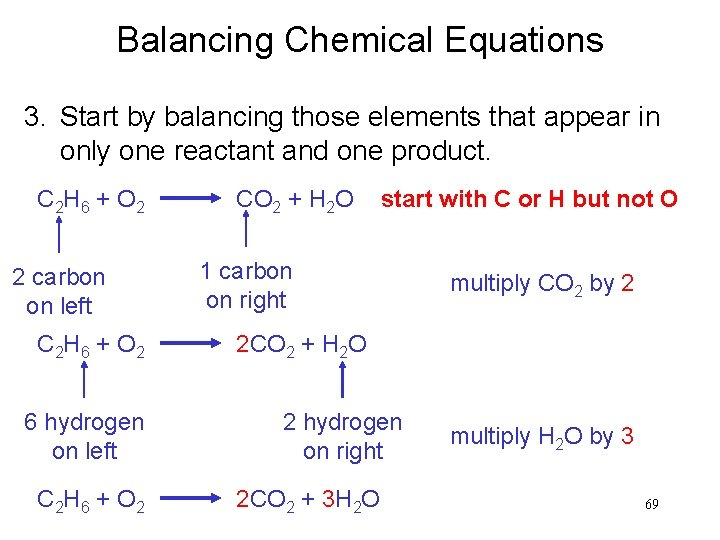Balancing Chemical Equations 3. Start by balancing those elements that appear in only one reactant and one product. C 2 H 6 + O 2 2 carbon on left C 2 H 6 + O 2 6 hydrogen on left C 2 H 6 + O 2 CO 2 + H 2 O start with C or H but not O 1 carbon on right multiply CO 2 by 2 2 CO 2 + H 2 O 2 hydrogen on right 2 CO 2 + 3 H 2 O multiply H 2 O by 3 69Balancing Chemical Equations 4. Balance those elements that appear in two or more reactants or products. C 2 H 6 + O 2 2 oxygen on left 2 CO 2 + 3 H 2 O multiply O 2 by 7 2 4 oxygen + 3 oxygen = 7 oxygen (3 x 1) on right (2 x 2) 7 C 2 H 6 + O 2 2 2 CO 2 + 3 H 2 O 2 C 2 H 6 + 7 O 2 4 CO 2 + 6 H 2 O remove fraction multiply both sides by 2 70Balancing Chemical Equations 5. Check to make sure that you have the same number of each type of atom on both sides of the equation. 2 C 2 H 6 + 7 O 2 4 CO 2 + 6 H 2 O 4 C (2 x 2) 4 C 12 H (2 x 6) 12 H (6 x 2) 14 O (7 x 2) 14 O (4 x 2 + 6) Reactants 4 C 12 H 14 O Products 4 C 12 H 14 O 71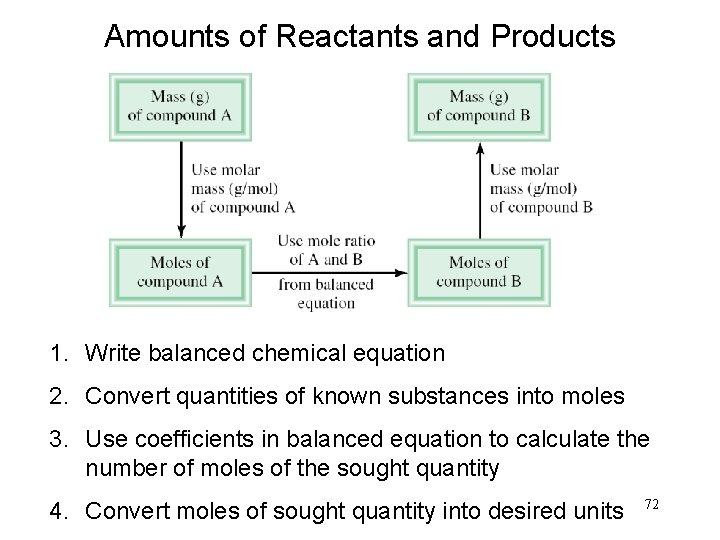Amounts of Reactants and Products 1. Write balanced chemical equation 2. Convert quantities of known substances into moles 3. Use coefficients in balanced equation to calculate the number of moles of the sought quantity 4. Convert moles of sought quantity into desired units 72Methanol burns in air according to the equation 2 CH 3 OH + 3 O 2 2 CO 2 + 4 H 2 O If 209 g of methanol are used up in the combustion, what mass of water is produced? grams CH 3 OH moles CH 3 OH molar mass CH 3 OH 209 g CH 3 OH x moles H 2 O grams H 2 O molar mass coefficients H 2 O chemical equation 4 mol H 2 O 18. 0 g H 2 O 1 mol CH 3 OH = x x 32. 0 g CH 3 OH 1 mol H 2 O 2 mol CH 3 OH 235 g H 2 O 73Limiting Reagent: Reactant used up first in the reaction. 2 NO + O 2 2 NO 2 NO is the limiting reagent O 2 is the excess reagent 74In one process, 124 g of Al are reacted with 601 g of Fe 2 O 3 2 Al + Fe 2 O 3 Al 2 O 3 + 2 Fe Calculate the mass of Al 2 O 3 formed. g Al g Fe 2 O 3 124 g Al x mol Al mol Fe 2 O 3 needed OR mol Al needed mol Fe 2 O 3 1 mol Al 27. 0 g Al x g Fe 2 O 3 needed 1 mol Fe 2 O 3 2 mol Al Start with 124 g Al 160. g Fe 2 O 3 = x 1 mol Fe 2 O 3 g Al needed 367 g Fe 2 O 3 need 367 g Fe 2 O 3 Have more Fe 2 O 3 (601 g) so Al is limiting reagent 75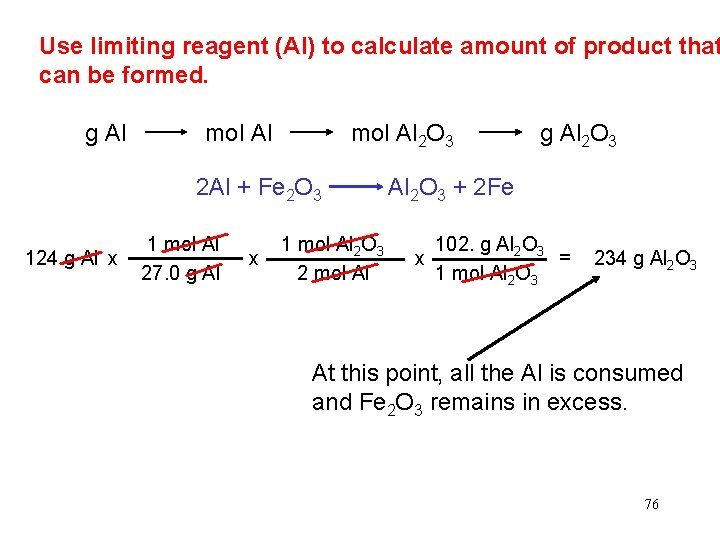Use limiting reagent (Al) to calculate amount of product that can be formed. g Al mol Al 2 O 3 g Al 2 O 3 2 Al + Fe 2 O 3 Al 2 O 3 + 2 Fe 124 g Al x 1 mol Al 27. 0 g Al x 1 mol Al 2 O 3 2 mol Al 102. g Al 2 O 3 = x 1 mol Al 2 O 3 234 g Al 2 O 3 At this point, all the Al is consumed and Fe 2 O 3 remains in excess. 76Reaction Yield Theoretical Yield is the amount of product that would result if all the limiting reagent reacted. Actual Yield is the amount of product actually obtained from a reaction. % Yield = Actual Yield Theoretical Yield x 100% 77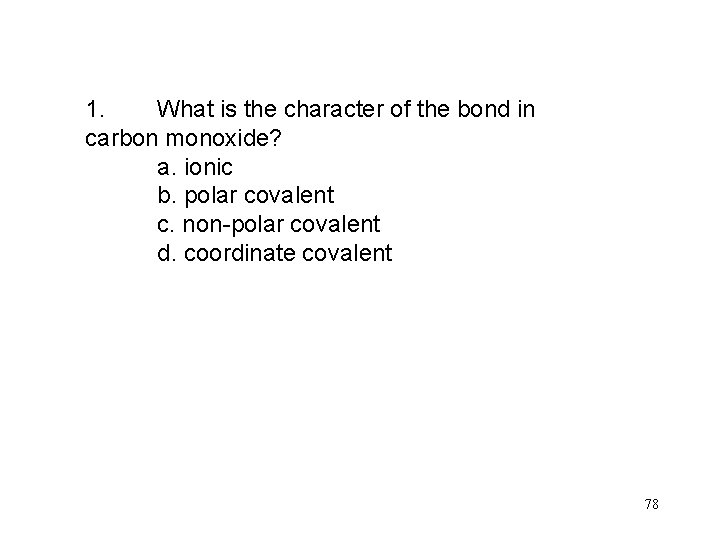1. What is the character of the bond in carbon monoxide? a. ionic b. polar covalent c. non-polar covalent d. coordinate covalent 78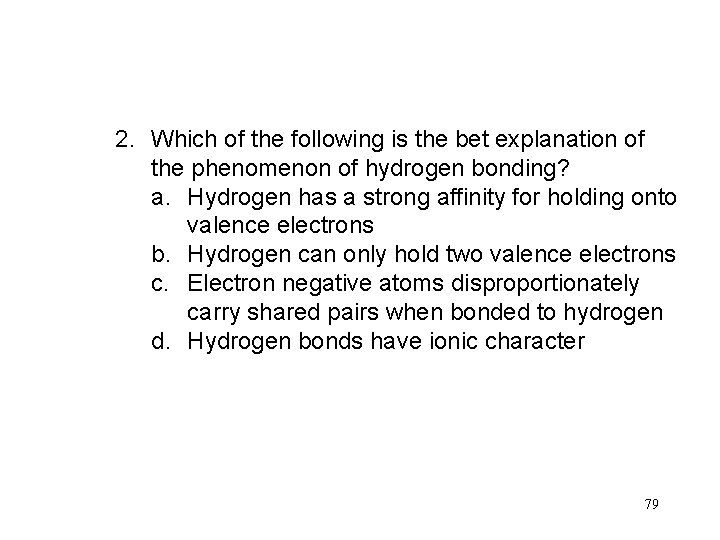2. Which of the following is the bet explanation of the phenomenon of hydrogen bonding? a. Hydrogen has a strong affinity for holding onto valence electrons b. Hydrogen can only hold two valence electrons c. Electron negative atoms disproportionately carry shared pairs when bonded to hydrogen d. Hydrogen bonds have ionic character 79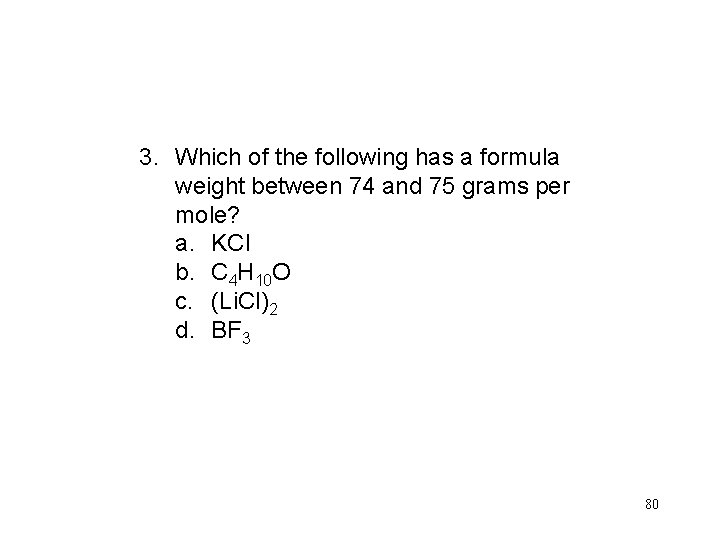3. Which of the following has a formula weight between 74 and 75 grams per mole? a. KCl b. C 4 H 10 O c. (Li. Cl)2 d. BF 3 804. In the reactoin shown, if 39. 03 g of Na 2 S are reacted with 113. 3 g of Ag. NO 3, how much, if any, of either reagent will be left over once the reaction has gone to completion? Na 2 S+ 2 Ag. NO 3 Ag 2 S + 2 Na. NO 3 a. b. c. d. 41. 37 g Ag. NO 3 13. 00 g Na 2 S 26. 00 g Na 2 S 74. 27 g Ag. NO 3 81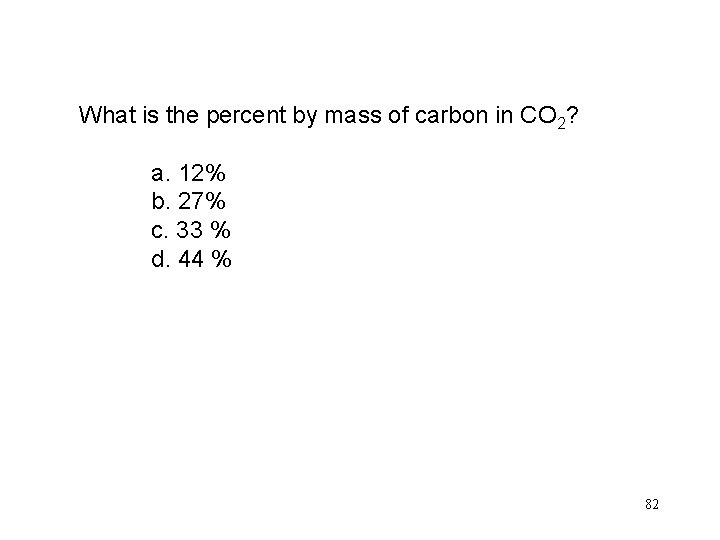What is the percent by mass of carbon in CO 2? a. 12% b. 27% c. 33 % d. 44 % 82Sulfur dioxide oxidizes in the presence of O 2 gas as per the reaction 2 SO 2 + O 2 2 SO 3 Approximately how many grams of sulfur trioxide are produced by the complete oxidation of 1 mole of sulfur dioxide? a. 1 g b. 2 g c. 80 g d. 160 g 83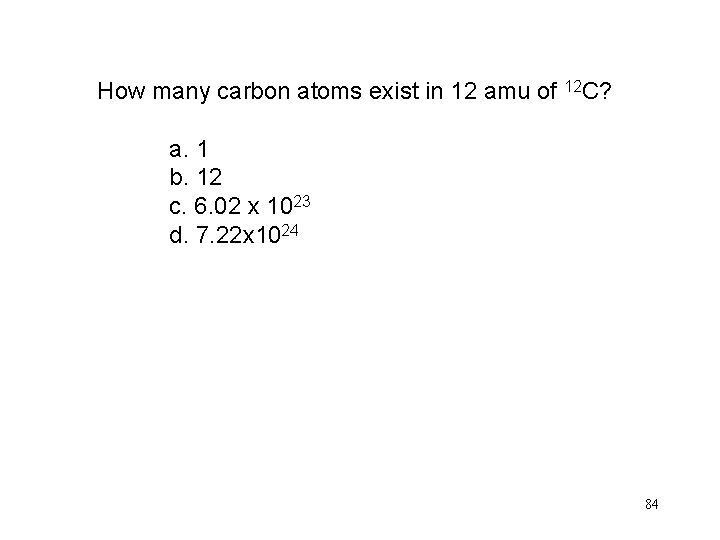How many carbon atoms exist in 12 amu of 12 C? a. 1 b. 12 c. 6. 02 x 1023 d. 7. 22 x 1024 84When an electron moves from a 2 p to a 3 s orbital, the atom containng that electron: a. becomes a new isotope b. becomes a new element c. absorbs energy d. releases energy 8586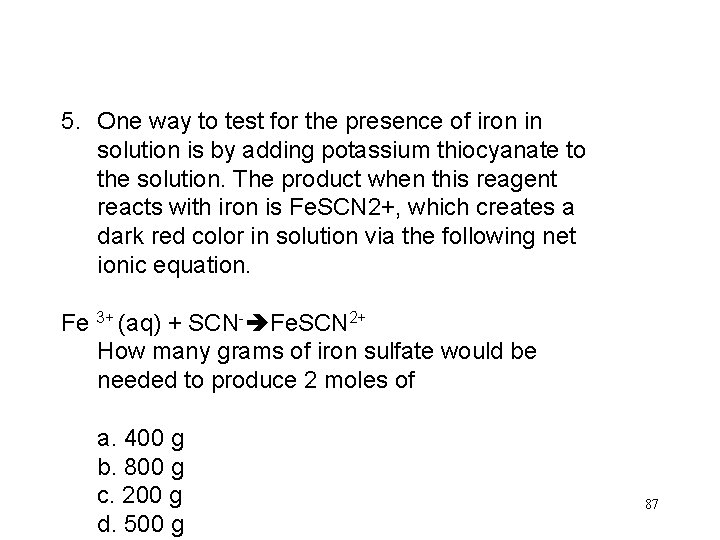5. One way to test for the presence of iron in solution is by adding potassium thiocyanate to the solution. The product when this reagent reacts with iron is Fe. SCN 2+, which creates a dark red color in solution via the following net ionic equation. Fe 3+ (aq) + SCN- Fe. SCN 2+ How many grams of iron sulfate would be needed to produce 2 moles of a. 400 g b. 800 g c. 200 g d. 500 g 87